﻿

On Mutually Orthogonal Disjoint Copies of Graph Squares

Abstract

A family of decompositions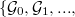$\{\mathcal{G}_{0},\mathcal{G}_{1},...,% \mathcal{G}_{k-1}\}$ of a complete bipartite graph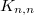$K_{n,n}$ is a set of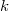$k$ \textit{mutually orthogonal graph squares}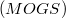$(MOGS)$ if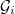$\mathcal{G}_{i}$ and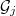$\mathcal{G}_{j}$ \ are orthogonal for all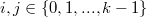$i,j\in \{0,1,...,k-1\}$ and$% i\neq j$. For any subgraph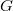$G$ of$K_{n,n}$ with$n$ edges,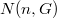$N(n,G)$ denotes the maximum number$k$ in a largest possible set(Error rendering LaTeX formula) of$(MOGS)$ of$K_{n,n}$ by$G$. Our objective of this paper is to compute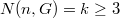$N(n,G)=k\geq 3$ where$G$ represents disjoint copies of certain subgraphs of$K_{n,n}$.

DOI Code: 10.1285/i15900932v36n2p89

Keywords: Orthogonal graph squares; Orthogonal double cover; Mutually orthogonal Latin squares

Full Text: PDF